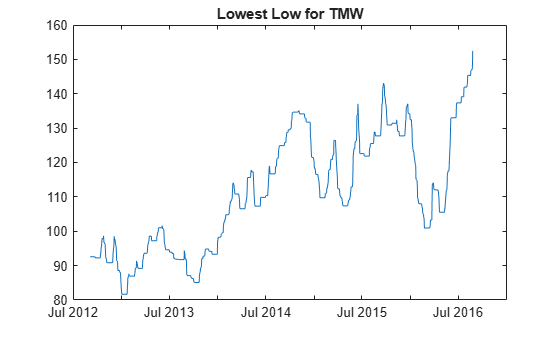# llow

## Syntax

``values = llow(Data)``
``values = llow(___,Name,Value)``

## Description

example

````values = llow(Data)` generates a vector of lowest low values from the series of low prices for the past n periods.```

example

````values = llow(___,Name,Value)` adds optional name-value pair arguments. ```

## Examples

collapse all

Load the file `SimulatedStock.mat`, which provides a timetable (`TMW`) for financial data for TMW stock.

```load SimulatedStock.mat values = llow(TMW); plot(values.Time,values.LowestLow) title('Lowest Low for TMW')```## Input Arguments

collapse all

Data for low prices, specified as a matrix, table, or timetable. Timetables and tables with `M` rows must contain a variable named `'Low'` (case insensitive).

Data Types: `double` | `table` | `timetable`

### Name-Value Arguments

Specify optional pairs of arguments as `Name1=Value1,...,NameN=ValueN`, where `Name` is the argument name and `Value` is the corresponding value. Name-value arguments must appear after other arguments, but the order of the pairs does not matter.

Before R2021a, use commas to separate each name and value, and enclose `Name` in quotes.

Example: `values = llow(TMW_LOW,'NumPeriods',10)`

Moving window for the lowest low calculation, specified as the comma-separated pair consisting of `'NumPeriods'` and a scalar positive integer.

Data Types: `double`

## Output Arguments

collapse all

Lowest low series, returned with the same number of rows (`M`) and the same type (matrix, table, or timetable) as the input `Data`.

 Achelis, S. B. Technical Analysis from A to Z. Second Edition. McGraw-Hill, 1995.